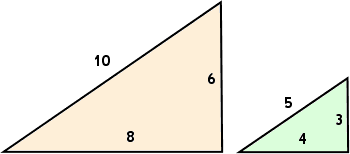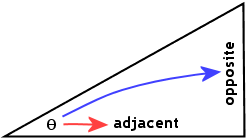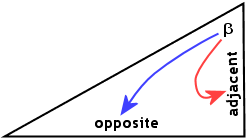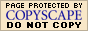Basic Trigonometric Ratios: Examples (page 1 of 2)Right triangles are nice and neat, with their side lengths obeying the Pythagorean Theorem. Any two right triangles with the same two non-right angles are "similar", in the technical sense that their corresponding sides are in proportion. For instance, the following two triangles (not drawn to scale) have all the same angles, so they are similar, and the corresponding pairs of their sides are in proportion:ratios of corresponding sides: 10/5 = 8/4 = 6/3 = 2
ratio of hypotenuse to base: 10/8 = 5/4 = 1.25
ratio of hypotenuse to height: 10/6 = 5/3 = 1.666...
ratio of height to base: 6/8 = 3/4 = 0.75

Around the fourth or fifth century AD, somebody very clever living in or around India noticed these consistency of the proportionalities of right triangles with the same sized base angles, and started working on tables of ratios corresponding to those non-right angles. There would be one set of ratios for the one-degree angle in a 1-89-90 triangle, another set of ratios for the two-degree angle in a 2-88-90 triangle, and so forth. These ratios are called the "trigonometric" ratios for a right triangle.

 Given a right triangle with a non-right angle designated as θ ("THAY-tuh"), we can label the hypotenuse (always the side opposite the right angle) and then label the other two sides "with respect to θ" (that is, in relation to the non-right angle θ that we're working with). The side opposite the angle θ is the "opposite" side, and the other side, being "next" to the angle (but not being the hypotenuse) is the "adjacent" side.     For the same triangle, if we called the third angle β ("BAY-tuh"), the labelling would be as shown: As you can see, the labels "opposite" and "adjacent" are relative to the angle in question.There are six ways to form ratios of the three sides of this triangle. I'll shorten the names from "hypotenuse", "adjacent", and "opposite" to "hyp", "adj", and "opp":

Each of these ratios has a name:

...and each of these names has an abbreviated notation, specifying the angle you're working with:

 name ratio notation sine opp/hyp sin(θ) cosine adj/hyp cos(θ) tangent opp/adj tan(θ)
 name ratio notation cosecant hyp/opp csc(θ) secant hyp/adj sec(θ) cotangent adj/opp cot(θ)

The ratios in the left-hand table are the "regular" trig ratios; the ones in the right-hand table are their reciprocals (that is, the inverted fractions). To remember the ratios for the regular trig functions, many students use the mnemonic SOH CAH TOA, pronounced "SOH-kuh-TOH-uh" (as though it's all one word). This mnemonic stands for:

• Sine is Opposite over Hypotenuse
• Cosine is Adjacent over Hypotenuse
• Tangent is Opposite over Adjacent

When two ratios have the same name other than the "co-" at the start of one of them, the pair are called "co-functions". (Note: The ratios didn't get their current names until the 12th century or so, as a result of Europeans making some mistakes when they translated Arabic texts.)